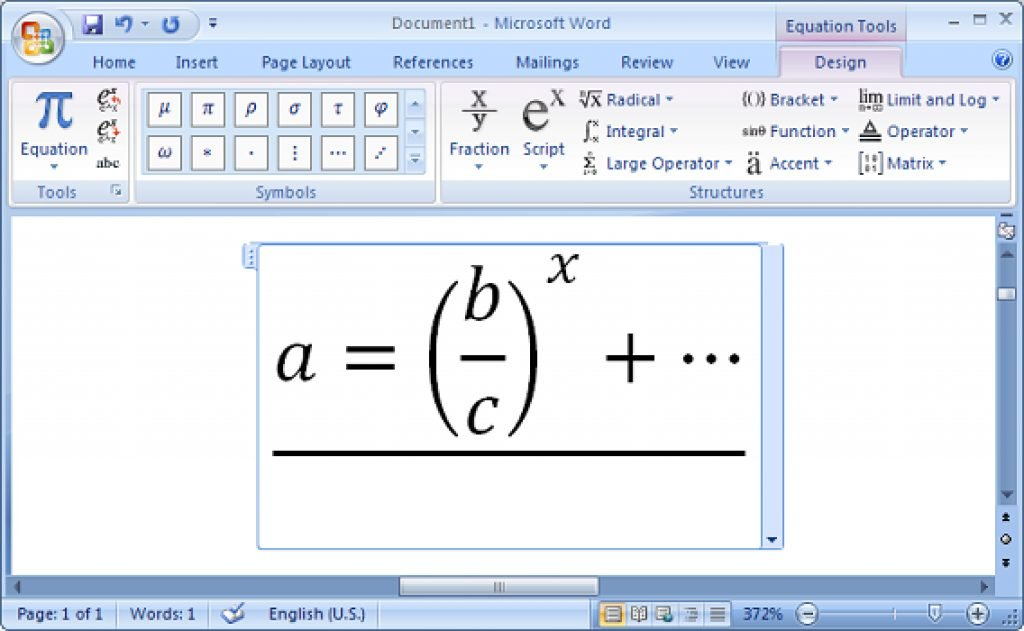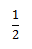Many of my friends asked me “How to use equation editor” in office-2010. Today I am going to show you the basics of using an equation editor. How to open it? How to use its many options, etc. Write Mathematical terms easily with “Equation Editor”

First of all, I’m not an Expert in Math, So I just want to show you how to use “Equation Editor”. Editing is your job; Please don’t mind !!

“Equation Editor”:-
Equation Editor is one of the best ways to write very important mathematical terms like Fraction, Radical, Script, Integral, Accent etc.

Suppose you want to write half. How does it looks if you write 1/2 like this?
With equation editor, you can easily do this.Your Writhing  should look like above

How to open Equation Editor in Word 2010?
In Microsoft Office, 2010, Everything is very nicely designed. To open Equation Editor, you have to first open Microsoft Office 2010 and have to go to the “Insert” option. Here you can see on the right side of the toolbar “Equation Editor”
Here is a snapshot to understand you:-

“Using Fraction”:-
The fraction option is using a divider between two words or the latter. Such as you want to write one and a half, a/b, y/x, ab/XY etc. Many mathematical terms like this can be easily written by this “Fraction” option.
I am giving you a snapshot of “Fraction” in Microsoft Office 2010.